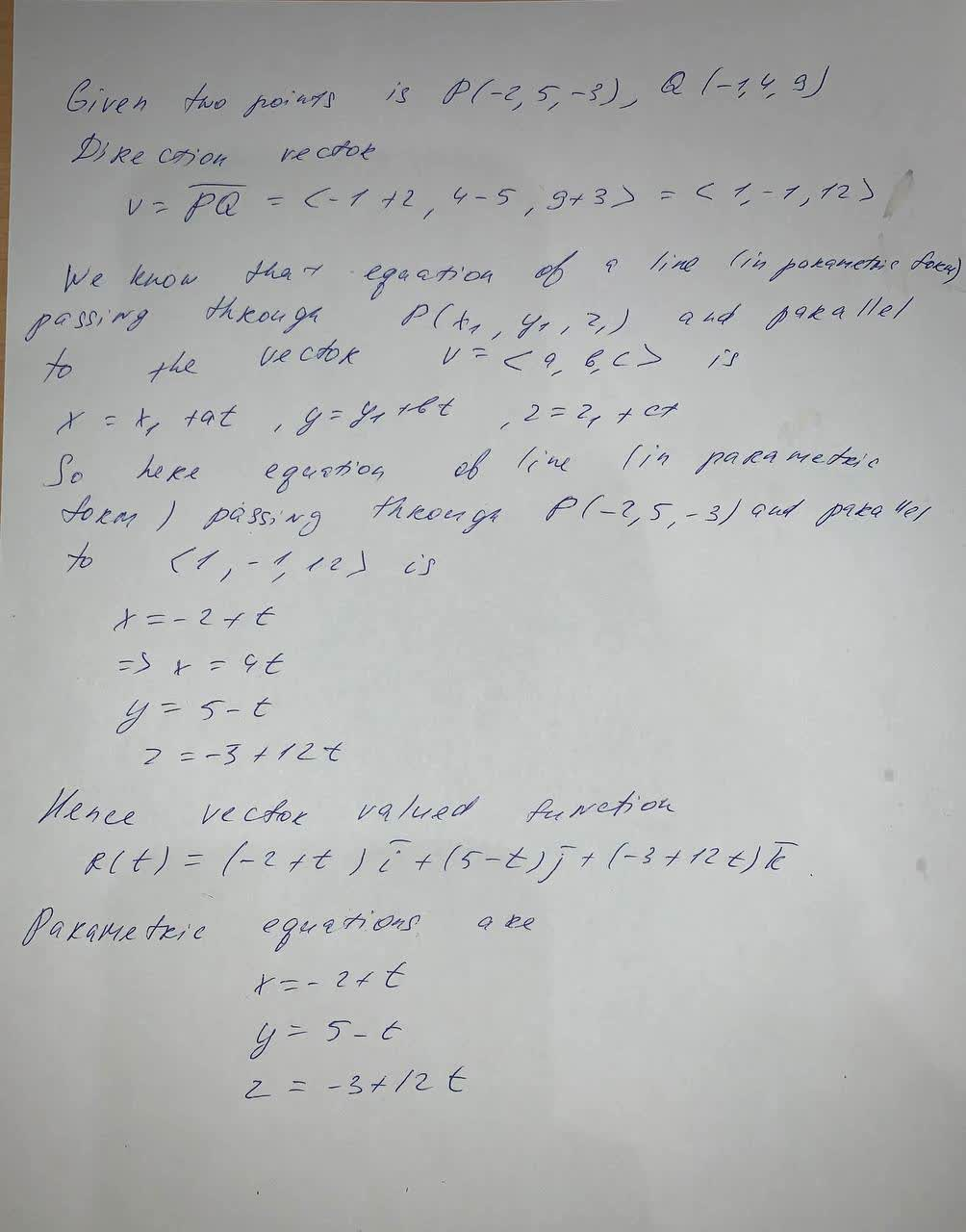rocedwrp

2021-08-29

Writing a Vector-Valued function in exercise , represent the line segment from P to Q by a vector-valued function and by a set of parametric equations:
P(-2,5,-3),Q(-1,4,9)To represent the line segment from P to Q by a vector-valued function you needDo you have a similar question?